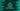# Java program to check if a string is a prime number or not## Java program to check if a string is a prime number or not:

In this post, we will learn how to check if a string is a prime number or not in Java. The program will take a string as input from the user, verify if it is a prime number string or not and print one result to the user.

We can use any loop to solve this problem. A number is called a prime number if it is greater than 1 and it is divisible by 1 and the number itself. If we can divide the number by any other number, it will not be a prime number.

### How to check if a number is prime or not programmatically:

If we want to check if a number is a prime number or not programmatically, we have to run one loop. The loop will run from 2 to number/2 and for each value, it will check if it can divide the number. If yes, it will not be a prime number. Else, it will be a prime number.

We can also run from 2 to the square root of the number. This will reduce the number of steps and for a large number, it will be faster than the previous method.

Let’s learn how to write these algorithms in Java :

### Method 1: By using a for loop:

We can use a for loop and run from 2 to number/2. On each iteration, it will check if any value can divide the number or not. If yes, it will not be a prime number.

We will take the value as a string and convert it to a number first.

``````import java.util.Scanner;

class Main {
public static boolean isPrime(String n) {
int number = Integer.parseInt(n);

if (number == 0 || number == 1) return false;

for (int i = 2; i <= number / 2; i++) {
if (number % i == 0) return false;
}
return true;
}

public static void main(String[] args) {
String num;

Scanner sc = new Scanner(System.in);
System.out.println("Enter the number: ");
num = sc.next();

if (isPrime(num)) {
System.out.println("It is a Prime number.");
} else {
System.out.println("It is not a Prime number.");
}
}
}``````

In this example,

• sc is a Scanner object to read the user input values.
• We are asking the user to enter the number. The number is stored in the string variable num.
• isPrime is a method to check if a string is prime or not. It takes a string value as its parameter and returns one boolean value.
• We are parsing the integer value at the start of this method and this value is stored in the variable number.
• If the value of a number is equal to 0 or 1, it returns false i.e. it is not a prime number.
• It runs one for loop from i = 2 to i = number/2 and for each value of i, it checks if it can divide the number or not. If yes, it returns false i.e. it is not a prime number.
• Once the loop ends, it returns true, i.e. the number is a prime number.

### Method 2: By using a while loop:

We can also use a while loop to write the same program. It will be similar to the above program. The only difference is that we will initialize a variable before the loop starts and the increment of i will be at the end of each iteration.

``````import java.util.Scanner;

class Main {
public static boolean isPrime(String n) {
int number = Integer.parseInt(n);

if (number == 0 || number == 1) return false;

int i = 2;

while (i <= number / 2) {
if (number % i == 0) return false;
i++;
}
return true;
}

public static void main(String[] args) {
String num;

Scanner sc = new Scanner(System.in);
System.out.println("Enter the number: ");
num = sc.next();

if (isPrime(num)) {
System.out.println("It is a Prime number.");
} else {
System.out.println("It is not a Prime number.");
}
}
}``````

### Method 3: By iterating up to the square root of the number:

We can also iterate from 2 to the square root of the number. It will give us the same result. The advantage of this approach is that the number of iterations will be reduced.

``````import java.util.Scanner;

class Main {
public static boolean isPrime(String n) {
int number = Integer.parseInt(n);

if (number == 0 || number == 1) return false;

int i = 2;

while (i <= Math.sqrt(number)) {
if (number % i == 0) return false;
i++;
}
return true;
}

public static void main(String[] args) {
String num;

Scanner sc = new Scanner(System.in);
System.out.println("Enter the number: ");
num = sc.next();

if (isPrime(num)) {
System.out.println("It is a Prime number.");
} else {
System.out.println("It is not a Prime number.");
}
}
}``````

To find the square root of the number, we are using Math.sqrt() method. If you run this program, it will give similar output.